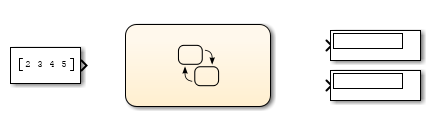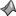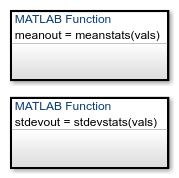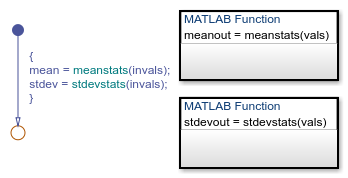## Program a MATLAB Function in a Chart

A MATLAB® function in a Stateflow® chart is a graphical element that you use to write algorithms that are easier to implement by calling built-in MATLAB functions. This type of function is useful for coding algorithms that are more easily expressed by using MATLAB instead of the graphical Stateflow constructs. For more information, see Reuse MATLAB Code by Defining MATLAB Functions.

Inside a MATLAB function, you can call these types of functions:

• Local functions defined in the body of the MATLAB function.

• Graphical, Simulink®, truth table, and other MATLAB functions in the chart.

• Built-in MATLAB functions that support code generation. These functions generate C code for building targets that conform to the memory and data type requirements of embedded environments.

• Extrinsic MATLAB functions that do not support code generation. These functions execute only in the MATLAB workspace during simulation of the model. For more information, see Call Extrinsic MATLAB Functions in Stateflow Charts.

• Simulink Design Verifier™ functions for property proving and test generation. These functions include:

This example shows how to create a model with a Stateflow chart that calls two MATLAB functions, `meanstats` and `stdevstats`:

• `meanstats` calculates the mean of the values in `vals`.

• `stdevstats` calculates a standard deviation for the values in `vals`.

### Build Model

1. Create a new model with the following blocks:2. Save the model as `call_stats_function_stateflow`.

3. In the model, double-click the Chart block.

4. In the object palette, use the MATLAB function iconto add two functions in the empty chart.

5. Label each function as shown:You must label a MATLAB function with its signature. Use the following syntax:

``[return_val1,return_val2,...] = function_name(arg1,arg2,...)``

You can specify multiple return values and multiple input arguments, as shown in the syntax. Each return value and input argument can be a scalar, vector, or matrix of values.

Tip

For MATLAB functions with only one return value, you can omit the brackets in the signature label.

6. In the chart, draw a default transition into a terminating junction with this condition action:

```{ mean = meanstats(invals); stdev = stdevstats(invals); }```

The chart should look something like this:Tip

If the formal arguments of a function signature are scalars, verify that inputs and outputs of function calls follow the rules of scalar expansion. For more information, see Assign Values to All Elements of a Matrix.

7. In the Modeling tab, under Design Data, select Model Explorer.

8. In the Model Hierarchy pane of the Model Explorer, select the function `meanstats`.The Contents pane displays the input argument `vals` and output argument `meanout`. Both are scalars of type `double` by default.

9. Double-click the `vals` row under the Size column to set the size of `vals` to `4`.

10. In the Model Hierarchy pane of the Model Explorer, select the function `stdevstats` and repeat the previous step.

11. In the Model Hierarchy pane of the Model Explorer, select `Chart` and add the following data:

Name

Scope

Size

`invals`

`Input`

4

`mean`

`Output`

Scalar (no change)

`stdev`

`Output`

Scalar (no change)

You should now see the following data in the Model Explorer.After you add the data `invals`, `mean`, and `stdev` to the chart, the corresponding input and output ports appear on the Stateflow block in the model.12. Connect the Constant and Display blocks to the ports of the Chart block and save the model.### Program MATLAB Functions

To program the functions `meanstats` and `stdevstats`, follow these steps:

1. Open the chart in the model `call_stats_function_stateflow`.

2. In the chart, open the function `meanstats`.

The function editor appears with the header:

`function meanout = meanstats(vals)`

This header is taken from the function label in the chart. You can edit the header directly in the editor, and your changes appear in the chart after you close the editor.

3. On the line after the function header, enter:

`%#codegen`

The `%#codegen` compilation directive helps detect compile-time violations of syntax and semantics in MATLAB functions supported for code generation.

4. Enter a line space and this comment:

`% Calculates the statistical mean for vals`

`len = length(vals);`

The function `length` is an example of a built-in MATLAB function that is supported for code generation. You can call this function directly to return the vector length of its argument `vals`. When you build a simulation target, the function length is implemented with generated C code. Functions supported for code generation appear in Functions and Objects Supported for C/C++ Code Generation (MATLAB Coder).

The variable `len` is an example of implicitly declared local data. It has the same size and type as the value assigned to it — the value returned by the function `length`, a scalar `double`. To learn more about declaring variables, see Numeric Types (MATLAB Coder).

The MATLAB function treats implicitly declared local data as temporary data, which exists only when the function is called and disappears when the function exits. You can declare local data for a MATLAB function in a chart to be persistent by using the `persistent` construct.

6. Enter this line to calculate the value of `meanout`:

`meanout = avg(vals,len);`

The function `meanstats` stores the mean of `vals` in the Stateflow data `meanout`. Because these data are defined for the parent Stateflow chart, you can use them directly in the MATLAB function.

Two-dimensional arrays with a single row or column of elements are treated as vectors or matrices in MATLAB functions. For example, in `meanstats`, the argument `vals` is a four-element vector. You can access the fourth element of this vector with the matrix notation `vals(4,1)` or the vector notation `vals(4)`.

The MATLAB function uses the functions `avg` and `sum` to compute the value of `mean`. `sum` is a function supported for code generation. `avg` is a local function that you define later. When resolving function names, MATLAB functions in your chart look for local functions first, followed by functions supported for code generation.

Note

If you call a function that the MATLAB function cannot resolve as a local function or a function for code generation, you must declare the function to be extrinsic.

7. Now enter this statement:

`coder.extrinsic("plot");`
8. Enter this line to plot the input values in `vals` against their vector index.

`plot(vals,"-+");`

Recall that you declared `plot` to be an extrinsic function because it is not supported for code generation. When the MATLAB function encounters an extrinsic function, it sends the call to the MATLAB workspace for execution during simulation.

9. Now, define the local function `avg`, as follows:

```function mean = avg(array,size) mean = sum(array)/size;```

The header for `avg` defines two arguments, `array` and `size`, and a single return value, `mean`. The local function `avg` calculates the average of the elements in `array` by dividing their sum by the value of argument `size`.

The complete code for the function `meanstats` looks like this:

```function meanout = meanstats(vals) %#codegen % Calculates the statistical mean for vals len = length(vals); meanout = avg(vals,len); coder.extrinsic("plot"); plot(vals,"-+"); function mean = avg(array,size) mean = sum(array)/size;```
10. Save the model.

11. Back in the chart, open the function `stdevstats` and add code to compute the standard deviation of the values in `vals`. The complete code should look like this:

```function stdevout = stdevstats(vals) %#codegen % Calculates the standard deviation for vals len = length(vals); stdevout = sqrt(sum(((vals-avg(vals,len)).^2))/len); function mean = avg(array,size) mean = sum(array)/size;```
12. Save the model again.